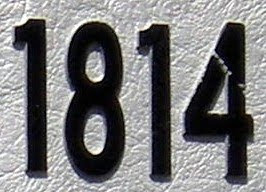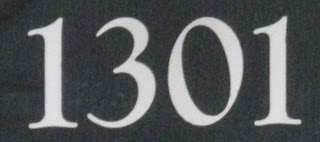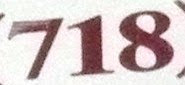## Thursday, December 24, 2009

### 2010

2010 = 2 x 3 x 5 x 67.

2010 is 133122 in base 4.

2010 is the solution to the postage stamp problem with 3 denominations and 30 stamps.

2010 is the maximal length of a rook's tour on a 14 x 15 chessboard.

2010 is a number having exactly four distinct prime factors, for which the largest is greater than the square root of the number.The 2010 Winter Olympic Games will be held in Vancouver, British Columbia, Feb. 12-28.

## Wednesday, December 23, 2009

### 2284

2284 = 22 x 571.

2284 is 203320 in base 4 and 4354 in base 8.

2284 is the number of 3 x 10 binary matrices.

2284 is the number of 7-digit perfect powers.

2284 is a year in which there will be five Fridays in February. It last happened in 2008 and will occur next in 2036.

## Tuesday, December 22, 2009

### 1900

1900 = 22 x 52 x 19.

1900 has the representation 1900 = 23 35 - 44.

There are 1900 prime numbers less than or equal to 215.

1900 is a 13-gonal (triskaeidecagonal or tridecagonal) number.

1900 is the sum of eight consecutive primes: 1900 = 223 + 227 + 229 + 233 + 239 + 241 + 251 + 257.

1900 is the largest palindrome in Roman numerals: MCM.

## Monday, December 21, 2009

### 1814

1814 = 2 x 907.

1814 is a number that is not the sum of a triangular number, a square, and a nonnegative cube.

1814 is a Fibonacci number (4181) written backwards.

1814 is 24224 in base 5, 12222 in base 6, and 2435 in base 9.George Stephenson designed his first locomotive in the year 1814.

## Friday, December 18, 2009

### 1504

1504 = 25 x 47.

1504 is an example of a number n for which 6n + 1 is a perfect square: 6(1504) + 1 = 9025 = 952.

1504 is the number of anisohedral polyiamonds with 21 cells. A tiling is isohedral if the symmetries of the tiling act transitively on the tiles; a shape is anisohedral if tiles the plane, but not isohedrally.

1504 is a number that is not the sum of a triangular number, a square, and a nonnegative cube. There are 714 such numbers below 2*109.On Feb. 29, 1504, Christopher Columbus used his knowledge of a lunar eclipse that night to persuade Jamaican tribesmen to provide him and his crew with supplies.

## Thursday, December 17, 2009

### 1412

1412 = 22 x 353.

1412 has a representation as a sum of two squares: 1412 = 162 + 342.

1412 is a number whose product of digits is equal to its sum of digits: 1 x 4 x 1 x 2 = 1 + 4 + 1 + 2 = 8.

1412 has a cube whose digits occur with the same frequency: 14123 = 2815166528. Each of its digits occur twice.St. Joan of Arc was born in the year 1412.

## Wednesday, December 16, 2009

### 1301

1301 is a prime number.

1301 has a representation as a sum of two squares: 1301 = 252 + 262.

1301 and 1303 form a twin prime pair.

1301 is the hypotenuse of a primitive Pythagorean triple: 13012 = 512 + 13002.

1301 can be represented as 24 34 + 5.Bromotrifluoromethane, also known by the trade name Halon 1301, is a colorless, odorless gas that was used to extinguish fires.

## Tuesday, December 15, 2009

### 1201

1201 is a prime number.

1201 has a representation as a sum of two squares: 1201 = 242 + 252.

1201 is the hypotenuse of a primitive Pythagorean triple: 12012 = 492 + 12002.

1201 has a square that is formed by inserted three 4s into it: 12012 = 1442401.The Apollo Guidance Computer showed a 1201 alarm (Executive overflow--no vacant areas) during Apollo 11's descent to the lunar surface.

## Monday, December 14, 2009

### 1115

1115 = 5 x 223.

1115 is the smallest four-digit integer, abcd, such that abcd/(a*b*c*d) is a prime number. Numbers that are divisible by the product of their digits are known as Zuckerman numbers to base 10.

1115 is the sum of nine consecutive prime numbers: 1115 = 103 + 107 + 109 + 113 + 127 + 131 + 139 + 149.

1115 is a multiple of 5 having only odd digits.

NGC 1115 is a galaxy in the constellation Aries.

## Friday, December 11, 2009

### 983

983 is a prime number.

983 is a number that cannot be written as a sum of three squares.

983 is the largest three-digit prime with distinct digits.

983 is a safe prime: a prime number of the form 2p + 1, where p is also a prime.983 is the number of words in U.S. President Theodore Roosevelt's Inaugural Address, delivered March 4, 1905.

## Thursday, December 10, 2009

### 517

517 = 11 x 47.

517 is the smallest number n such that n + sigma(n) + sigma(sigma(n)) is a cube, where sigma(n) is the sum of all divisors of n.

517 is the sum of five consecutive prime numbers: 517 = 97 + 101 + 103 + 107 + 109.

517 is a Smith number. A Smith number is a composite number for which, in a given base, the sum of its digits is equal to the sum of the digits in its prime factorization: 5 + 1 + 7 = 1 + 1 + 4 + 7.517 is the telephone area code that covers Lansing, Mich., and neighboring areas.

## Wednesday, December 9, 2009

### 669

669 = 3 x 223.

669 is the number of unsymmetrical ways to dissect a regular 12-gon into 10 triangles.

669 is 3033 in base 6 and 1235 in base 8.

669 is the number of primes less than 5,000.NGC 669 is a galaxy in the constellation Triangulum.

## Tuesday, December 8, 2009

### 425

425 = 52 x 17.

425 has three representations as a sum of two squares: 425 = 52 + 202 = 82 + 192 = 132 + 162.

425 is the hypotenuse of two primitive Pythagorean triples: 4252 = 872 + 4162 = 2972 + 3042.

425 is a pentagonal number, a number of the form n(3n - 1)/2, where n = 17.

425 is the number of subsets of {1, 2, 3, . . . 11} that have an integer average.425 is the telephone area code for suburbs to the north and east of Seattle, Washington.

## Monday, December 7, 2009

### 384

384 = 27 x 3.

384 has a unique representation as the sum of three squares: 384 = 82 + 82 + 162.

2*3842 - 1 and 384*2384 - 1 are both prime numbers.

384 is the sum of two successive primes: 191 + 193 = 384.A Chinese lunar leap year may have 384 full days.

## Friday, December 4, 2009

### 283

283 is a prime number.

281 and 283 form a twin prime pair.

283 = (6! - 5! - 4! - 3! - 2! - 1! - 0!)/2.

283 is the smallest prime number that is less than the sum of the cube roots of all primes less than itself.

## Thursday, December 3, 2009

### 142

142 = 2 x 71.

142 has the unique representation 142 = 52 + 62 + 92 as the sum of three squares.

142 is the smallest semiprime (having exactly two prime factors) whose sum of divisors is a cube: 1 + 2 + 71 + 142 = 216.

142 is the number of integer partitions of 25 into distinct parts.

142 is the number of planar graphs with six vertices.

Cartoon character Bugs Bunny once correctly calculated 1297 times 142 in his head and said, "If there's one thing we wabbits can do, it's multiply."

Source: Number Gossip

## Wednesday, December 2, 2009

### 881

881 is a prime number.

881 has a representation as the sum of two squares: 162 + 252.

881 and 883 form a twin prime pair.

881 is the hypotenuse of a primitive Pythagorean triple: 8812 = 3692 + 8002.

881 is the largest three-digit prime of any form ABC such that AB + C, A + BC, and A + B + C are prime: 88 + 1 = 89, 8 + 81 = 89, and 8 + 8 + 1 = 17.
881 is a 2007 Singaporean musical-comedy-drama film written and directed by Royston Tan.

## Tuesday, December 1, 2009

### 718

718 = 2 x 359. It is the product of two distinct Sophie Germain primes.

718 is the number of unlabeled topologies (topological spaces) with six elements.

718 is 23032 in base 4.

The sum of the even digits of 718 equals the sum of the odd digits: 7 + 1 = 8.718 is a New York City telephone area code.